## Data on holdover time of lightning-ignited wildfires

### Basic information

Author: Jose V. Moris

Contact:

Affiliation: DISAFA, University of Turin (Italy)

Date: November 2022

R version: 4.1.0

RStudio Desktop version: 1.4.1106

Platform: Windows 10 64-bit

First load some necessary libraries. Then load the data and get some information. Make sure that the 3 csv files containing the data are located in the working directory.

# load libraries
library(tidyverse) # version 1.3.1
library(knitr) # version 1.33
# load the data
CD <- read.csv("1_censored_data.csv") # censored data
NCD <- read.csv("2_non_censored_data.csv") # non-censored data

# check
class(CD)
##  "data.frame"
names(CD)
##   "Study_id"          "Reference"         "Time_interval"
##   "Time_interval_d"   "Lower_limit_d"     "Upper_limit_d"
##   "N_fires"           "RF"                "CRF"
##  "Original_data"     "Data_location"     "Collection_method"
str(CD)
## 'data.frame':    2311 obs. of  12 variables:
##  $Study_id : chr "CON2006CH01" "CON2006CH01" "CON2006CH01" "CON2006CH01" ... ##$ Reference        : chr  "Conedera et al 2006" "Conedera et al 2006" "Conedera et al 2006" "Conedera et al 2006" ...
##  $Time_interval : chr "1 day" "1 day" "1 day" "1 day" ... ##$ Time_interval_d  : num  1 1 1 1 1 1 1 1 1 1 ...
##  $Lower_limit_d : num 0 1 2 3 4 5 6 0 1 2 ... ##$ Upper_limit_d    : num  1 2 3 4 5 6 7 1 2 3 ...
##  $N_fires : int 117 28 4 1 2 1 1 15 6 1 ... ##$ RF               : num  0.75974 0.18182 0.02597 0.00649 0.01299 ...
##  $CRF : num 0.76 0.942 0.968 0.974 0.987 ... ##$ Original_data    : chr  "N" "N" "N" "N" ...
##  $Data_location : chr "Figure 4" "Figure 4" "Figure 4" "Figure 4" ... ##$ Collection_method: chr  "Personal communication" "Personal communication" "Personal communication" "Personal communication" ...
dim(CD)
##  2311   12

## Censored data

### Plots

Create some barplots to observe the distributions of holdover time. We are using barplots because the censored data consist of frequency distributions.

# barplots
bp1 <- CD %>% filter(Study_id %in% c("CON2006CH01")) # choose one study

ggplot(bp1, aes(x = factor(round(Upper_limit_d, 2)), y = RF*100)) + # barplot
geom_bar(stat="identity") +
labs(x = "Holdover time (days)", y = "Relative frequency of LIW (%)")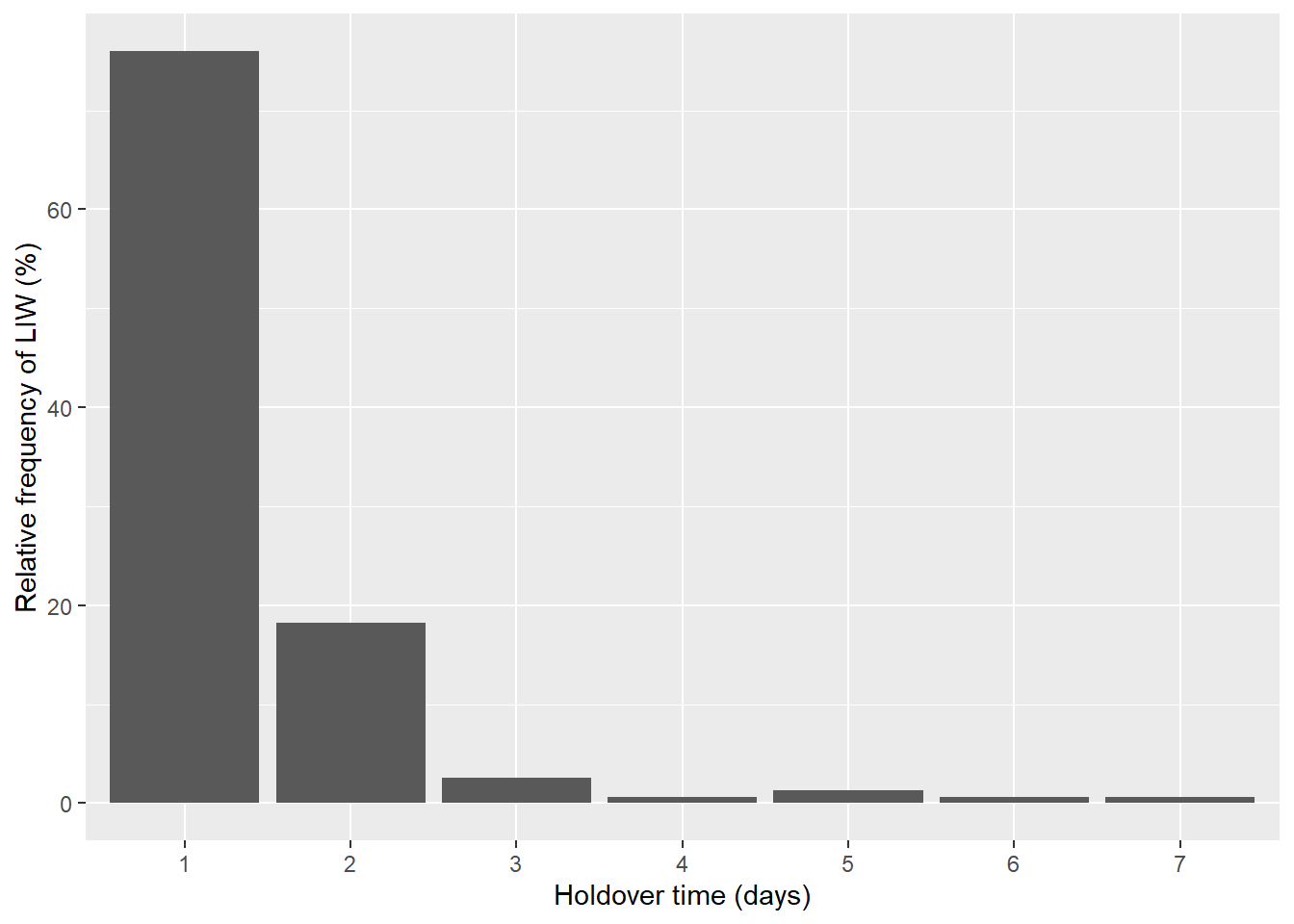bp2 <- CD %>% filter(Study_id %in% c("KOU1967CA01")) # choose one study

ggplot(bp2, aes(x = factor(round(Upper_limit_d, 2)), y = RF*100)) + # barplot
geom_bar(stat="identity") +
labs(x = "Holdover time (days)", y = "Relative frequency of LIW (%)")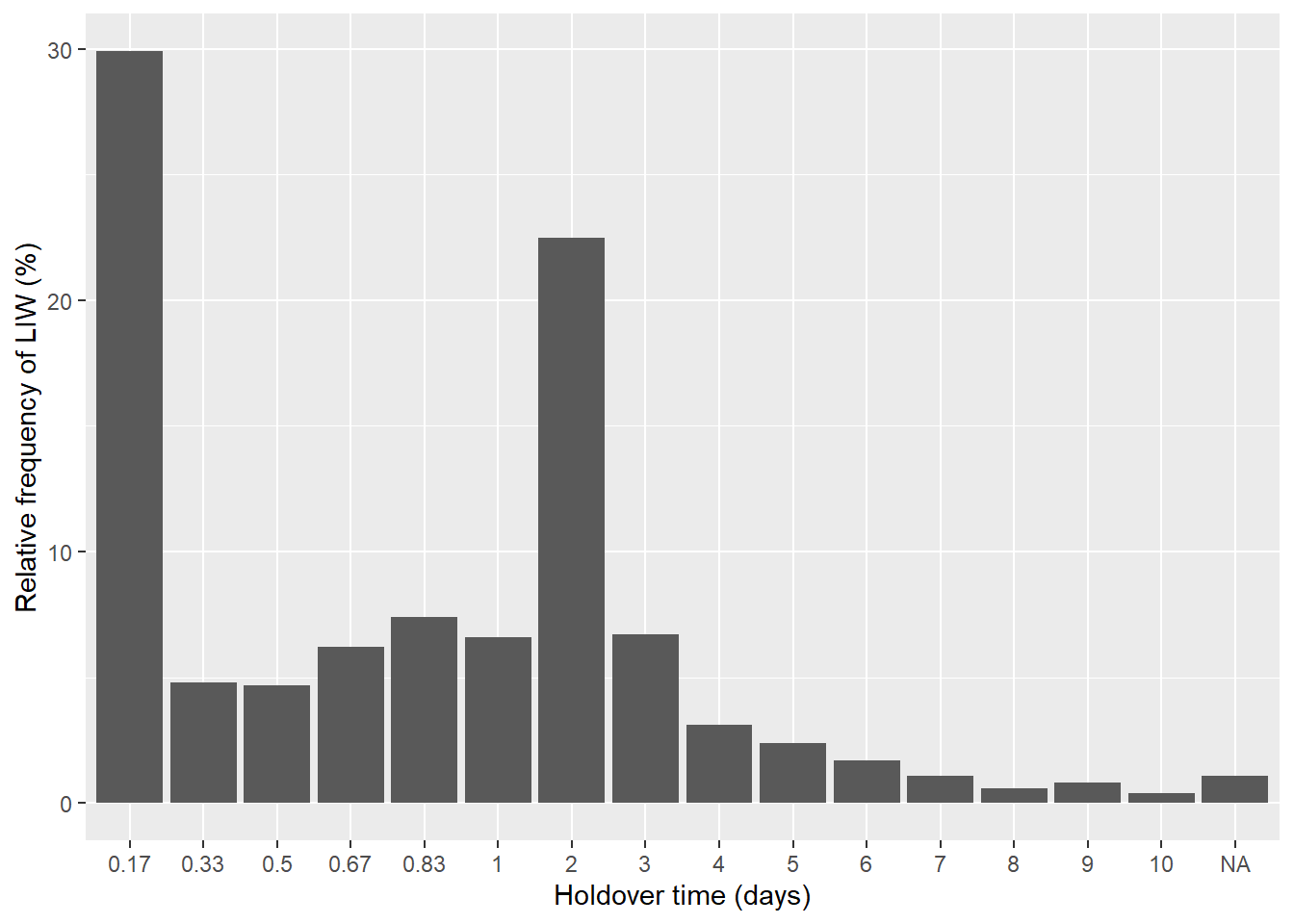bp3 <- CD %>% filter(Study_id %in% c("DOR2022AU01")) # choose one study

ggplot(bp3, aes(x = Upper_limit_d, y = RF*100)) + # barplot
geom_bar(stat="identity") +
labs(x = "Holdover time (days)", y = "Relative frequency of LIW (%)")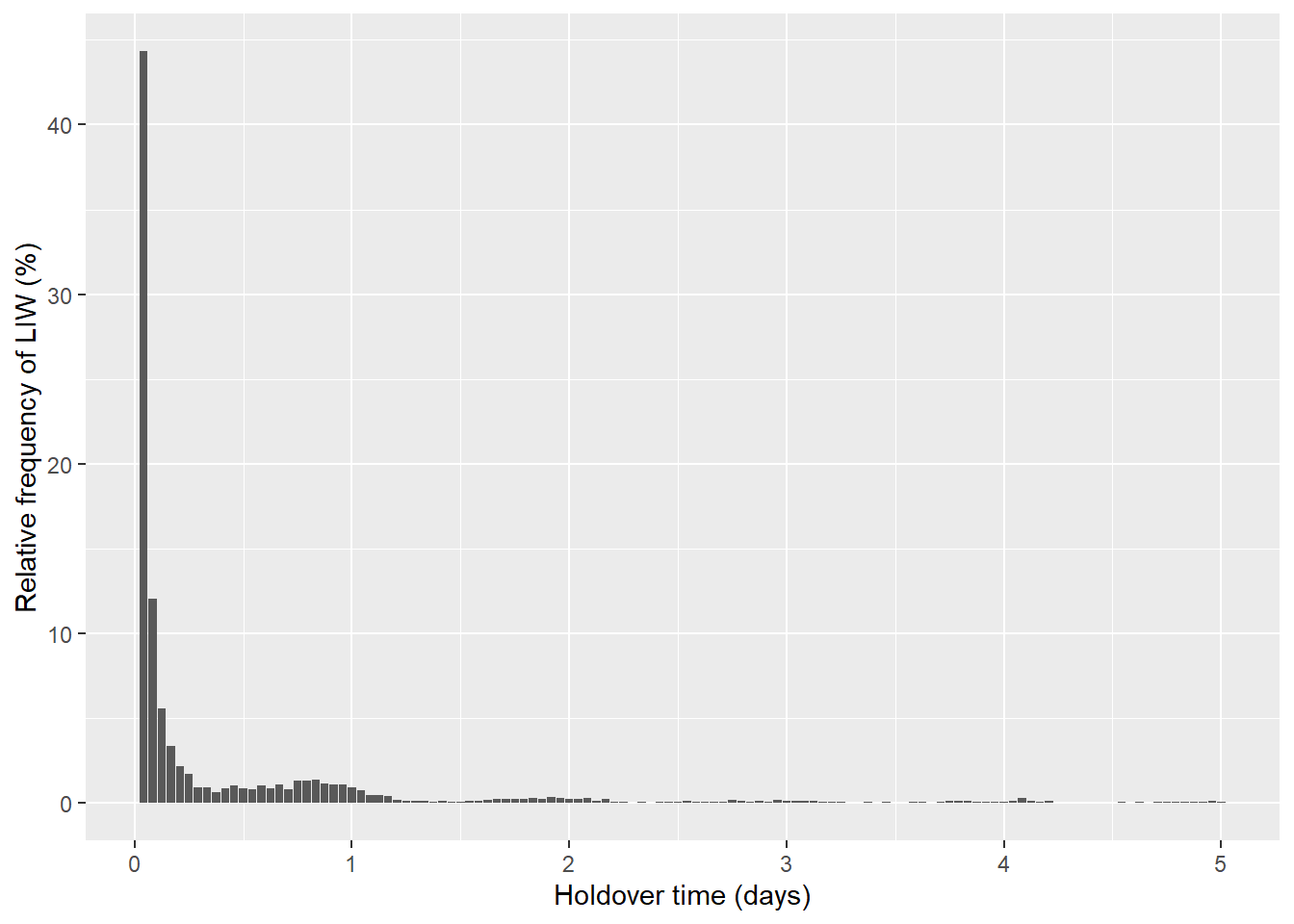bp <- CD %>% filter(Study_id %in% c("CON2006CH01", # choose several studies
"KOU1967CA01",
"PIN2017ES01",
"DOR2022AU01")) %>%
mutate(day = cut(Upper_limit_d, # calculate daily relative frequency
seq(0, max(Upper_limit_d, na.rm = T) +1))) %>%
group_by(Study_id, day) %>%
summarize(RF = sum(RF))

bp %>% group_by(Study_id) %>%
summarize(check = sum(RF)) # the values must be 1
## # A tibble: 4 x 2
##   Study_id    check
##   <chr>       <dbl>
## 1 CON2006CH01  1.00
## 2 DOR2022AU01  1.00
## 3 KOU1967CA01  1
## 4 PIN2017ES01  1.00
ggplot(bp, aes(x = day, y = RF*100)) + # barplot with several distributions
geom_bar(stat="identity") +
labs(x = "Holdover time (days)", y = "Relative frequency of LIW (%)") +
geom_text(aes(label = round(RF*100, 1), vjust = -0.25)) +
ylim(0,100) +
facet_wrap(~Study_id, ncol = 1)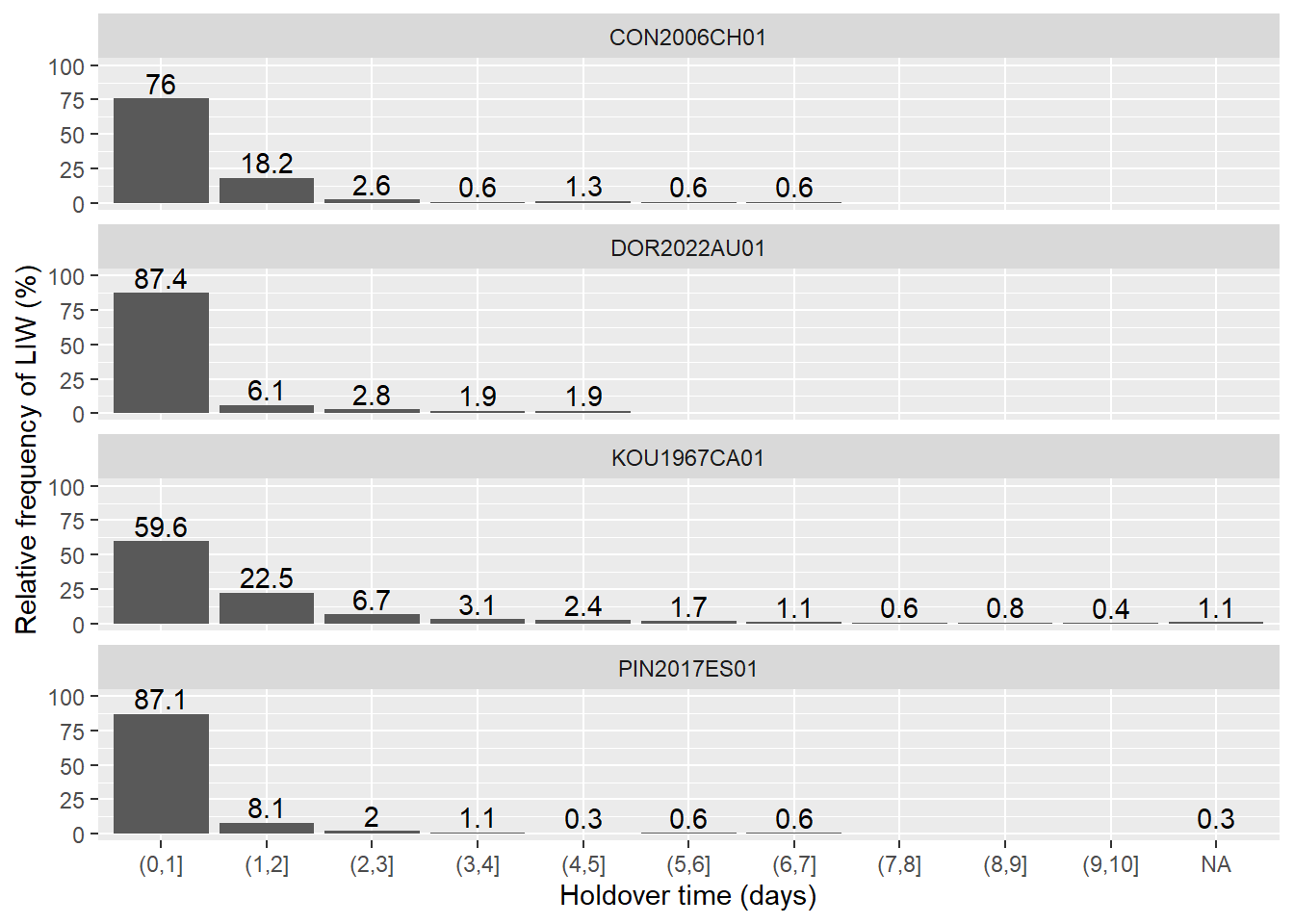### Statistics

Calculate some summary statistics using the frequency distributions of holdover time. Note that some values may be missing due to the nature of the censored data.

# calculate the mean and maximum HOT (holdover time)
cd_mean <- CD %>% group_by(Study_id) %>%
summarize(mean = sum((Lower_limit_d + Time_interval_d/2)*RF)*24, # mean HOT in hours
max = max(Upper_limit_d)) %>% # maximum HOT in days
select(Study_id, mean, max)

# calculate the median HOT
cd_median <- CD %>% mutate(a = CRF - 0.5, # create a secondary variable
a = ifelse(a < 0, 100, a)) %>%
group_by(Study_id) %>%
filter(a == min(a)) %>% # find the interval in which the median value is
filter(Lower_limit_d == min(Lower_limit_d)) %>% # in case repeated values exist
mutate(median = (Lower_limit_d + ( 0.5 - (CRF-RF) )/RF*Time_interval_d)*24) %>% # median HOT in hours
select(Study_id, median)

# calculate the cumulative relative frequency (CRF) of HOT of day 1
cd_crf_d1 <- CD %>% filter(Upper_limit_d==1) %>%
rename(crf_d1 = CRF) %>%
mutate(crf_d1 = crf_d1*100) %>% # CRF of HOT of the first day in percentage
select(Study_id, crf_d1)

# join all the statistics
cd_sta <- cd_mean %>% left_join(cd_median, by = "Study_id") %>%
left_join(cd_crf_d1, by = "Study_id") %>%
rename(mean_h = mean,
max_d = max,
median_h = median,
crf_d1_pct = crf_d1)

kable(cd_sta, caption = "Summary statistics of censored data") # table
Summary statistics of censored data
Study_id mean_h max_d median_h crf_d1_pct
BAR1951US01 NA NA 4.158568 78.99560
BAR1978US01 NA NA 2.990812 90.17000
CON2006CH01 21.038962 7.000000 15.794872 75.97403
CON2006IT01 30.240000 6.000000 20.000000 60.00000
DOR2022AU01 11.031065 5.000000 1.471201 87.39855
DOW2009AU01 320.968280 90.000000 18.541702 64.71898
DUN2010US01 146.400000 23.000000 23.389830 51.30435
GIS1926US01 NA NA 4.750000 85.00000
GIS1931US01 NA NA 4.000000 86.00000
HES2022CA01 51.665454 5.000000 47.680000 23.09091
HES2022US01 44.597015 5.000000 38.550000 25.87065
HES2022US02 41.686411 5.000000 37.043478 28.22300
KOU1967CA01 NA NA 18.378379 59.60000
LAR2005FI01 NA NA 34.666667 42.45283
MAC2019US01 72.336000 11.000000 45.438735 27.40000
MEN2022BR01 22.911318 2.708333 21.595238 61.50943
MOR1948US01 NA NA 6.420601 78.49000
MOR2020CH01 30.777557 9.916667 13.125001 63.11787
MOR2020IT01 26.718750 6.250000 6.000000 71.87500
MUL2021AT01 33.940594 10.000000 17.911330 66.99670
NAS1996CA01 61.627601 15.000000 27.251799 47.78518
PER2021ES01 37.897135 14.000000 5.709677 72.79793
PER2021FR01 37.305558 10.083333 3.000000 75.00000
PER2021GR01 33.829320 3.958333 29.200000 43.43545
PER2021PT01 23.004858 3.875000 15.928572 64.07767
PER2022US01 20.769134 7.000000 12.054688 75.92446
PER2022US02 21.541219 6.958333 13.107143 74.34088
PIN2014ES01 8.597668 3.000000 1.409283 NA
PIN2017ES01 NA NA 1.611111 87.11485
PIN2022ES01 14.271973 9.708333 1.833333 84.40276
SCH2019US01 39.041412 15.000000 17.645756 68.00502
SHO1923US01 NA NA 15.257143 67.00000
SHO1930US01 NA NA 12.827586 68.00000
TAY1969US01 NA NA 10.169491 77.00000
WOT2005CA01 85.945445 28.000000 44.549098 33.46876
WOT2022CA01 88.944727 22.000000 21.186312 56.64035
WOT2022CA02 81.169302 22.000000 19.001683 63.15230
WOT2022CA03 52.845977 22.000000 18.052219 66.47382
WOT2022CA04 59.750589 22.000000 24.905222 49.34630
WOT2022CA05 75.351697 22.000000 39.796767 38.78836
WOT2022CA06 82.683820 22.000000 53.602617 25.53404
XUW2022RU01 47.348838 8.000000 38.692683 30.54264

## Non-censored data

### Statistics

Calculate some summary statistics using non-censored data of holdover time. Note that the data may need some manipulation before being analyzed.

# check
class(NCD)
##  "data.frame"
names(NCD)
##  "MOR2020IT01" "MOR2020CH01" "PER2021FR01" "PER2021PT01" "PER2021ES01"
##  "MEN2022BR01" "PER2022US01" "PER2022US02" "PIN2022ES01"
str(NCD)
## 'data.frame':    6301 obs. of  9 variables:
##  $MOR2020IT01: num 0.628 0.837 1.194 1.79 2.159 ... ##$ MOR2020CH01: num  0.0181 0.0353 0.0769 0.0928 0.0986 ...
##  $PER2021FR01: num 0.133 0.217 0.233 0.25 0.483 ... ##$ PER2021PT01: num  0.0167 0.0167 0.0667 0.0667 0.0667 ...
##  $PER2021ES01: num 0 0 0 0 0 0 0 0 0 0 ... ##$ MEN2022BR01: num  0.0167 0.1592 0.2658 0.3611 0.4486 ...
##  $PER2022US01: num 0.000556 0.002778 0.003056 0.004444 0.005 ... ##$ PER2022US02: num  0 0.000278 0.001667 0.002222 0.003333 ...
##  $PIN2022ES01: num 0.01 0.01 0.01 0.01 0.01 0.01 0.01 0.01 0.01 0.01 ... dim(NCD) ##  6301 9 # an option to manipulate non-censored data ncd <- as.list(NCD) # from dataframe to list ncd <- lapply(ncd, na.omit) # remove NA values # some statistics lapply(ncd, length) ##$MOR2020IT01
##  32
##
## $MOR2020CH01 ##  263 ## ##$PER2021FR01
##  36
##
## $PER2021PT01 ##  309 ## ##$PER2021ES01
##  2702
##
## $MEN2022BR01 ##  265 ## ##$PER2022US01
##  6301
##
## $PER2022US02 ##  2693 ## ##$PIN2022ES01
##  1013
sum(unlist(lapply(ncd, length))) # 13,614 fires
##  13614
lapply(ncd, mean)
## $MOR2020IT01 ##  26.69755 ## ##$MOR2020CH01
##  30.77072
##
## $PER2021FR01 ##  37.3287 ## ##$PER2021PT01
##  22.98403
##
## $PER2021ES01 ##  37.83713 ## ##$MEN2022BR01
##  22.86785
##
## $PER2022US01 ##  20.75002 ## ##$PER2022US02
##  21.5038
##
## $PIN2022ES01 ##  14.17972 lapply(ncd, median) ##$MOR2020IT01
##  6.275556
##
## $MOR2020CH01 ##  13.00722 ## ##$PER2021FR01
##  3.716667
##
## $PER2021PT01 ##  15.55 ## ##$PER2021ES01
##  5.725
##
## $MEN2022BR01 ##  21.65639 ## ##$PER2022US01
##  12.10611
##
## $PER2022US02 ##  13.08028 ## ##$PIN2022ES01
##  1.7
lapply(ncd, min)
## $MOR2020IT01 ##  0.6283333 ## ##$MOR2020CH01
##  0.01805556
##
## $PER2021FR01 ##  0.1333333 ## ##$PER2021PT01
##  0.01666667
##
## $PER2021ES01 ##  0 ## ##$MEN2022BR01
##  0.01666667
##
## $PER2022US01 ##  0.00055556 ## ##$PER2022US02
##  0
##
## $PIN2022ES01 ##  0.01 lapply(ncd, max) ##$MOR2020IT01
##  149.1961
##
## $MOR2020CH01 ##  237.3253 ## ##$PER2021FR01
##  241.85
##
## $PER2021PT01 ##  92.38333 ## ##$PER2021ES01
##  335.6667
##
## $MEN2022BR01 ##  64.43639 ## ##$PER2022US01
##  167.8397
##
## $PER2022US02 ##  166.8367 ## ##$PIN2022ES01
##  232.9333
# another option to manipulate non-censored data
ncd2 <-data.frame(unlist(ncd)) # from list to dataframe
ncd2 <- rownames_to_column(ncd2, "Study_id") %>% # rename variables
rename(HOT = unlist.ncd.) %>%
mutate(Study_id = substr(Study_id, 1, 11)) # keep the Study_id variable correct
dim(ncd2)
##  13614     2
# additional statistics
ncd_sta <- ncd2 %>% group_by(Study_id) %>% summarize(
n = n(), # number fires
median_h = median(HOT), # median HOT in hours
mean_h = mean(HOT), # mean HOT in hours
min_min = min(HOT)*60, # minimum HOT in minutes
max_d = max(HOT)/24, # maximum HOT in days
crf_d1_pct = sum(HOT <= 24) / n *100, # CRF of HOT of the firs day in percentage
crf_d3_pct = sum(HOT <= 72) / n *100) # CRF of HOT of the first 3 days in percentage

kable(ncd_sta, caption = "Summary statistics of non-censored data") # table
Summary statistics of non-censored data
Study_id n median_h mean_h min_min max_d crf_d1_pct crf_d3_pct
MEN2022BR01 265 21.656389 22.86785 1.0000002 2.684849 61.50943 100.00000
MOR2020CH01 263 13.007222 30.77072 1.0833336 9.888553 63.11787 87.45247
MOR2020IT01 32 6.275556 26.69755 37.6999998 6.216505 71.87500 84.37500
PER2021ES01 2702 5.725000 37.83713 0.0000000 13.986111 72.79793 84.08586
PER2021FR01 36 3.716667 37.32870 7.9999998 10.077083 75.00000 80.55556
PER2021PT01 309 15.550000 22.98403 1.0000002 3.849306 64.07767 96.76375
PER2022US01 6301 12.106111 20.75002 0.0333336 6.993322 75.92446 93.49310
PER2022US02 2693 13.080278 21.50380 0.0000000 6.951528 74.34088 92.38767
PIN2022ES01 1013 1.700000 14.17972 0.6000000 9.705556 84.40276 96.34748

### Plots

Create some histograms to observe the distributions of holdover time. We are using histograms because the non-censored data consist of estimated continuous values of holdover time (in hours) without any censoring.

# histograms
h1 <- ncd2 %>% filter(Study_id %in% c("PER2022US01")) # choose one study

ggplot(h1, aes(x=HOT, y=..count../sum(..count..)*100)) + # hourly histogram
geom_histogram(colour="black", boundary = 0, binwidth = 1) +
scale_x_continuous(breaks=c(0, 24, 48, 72, 96, 120, 144, 168)) +
labs(x = "Holdover time (hours)", y = "Relative frequency of LIW (%)") +
ggtitle(h1$Study_id)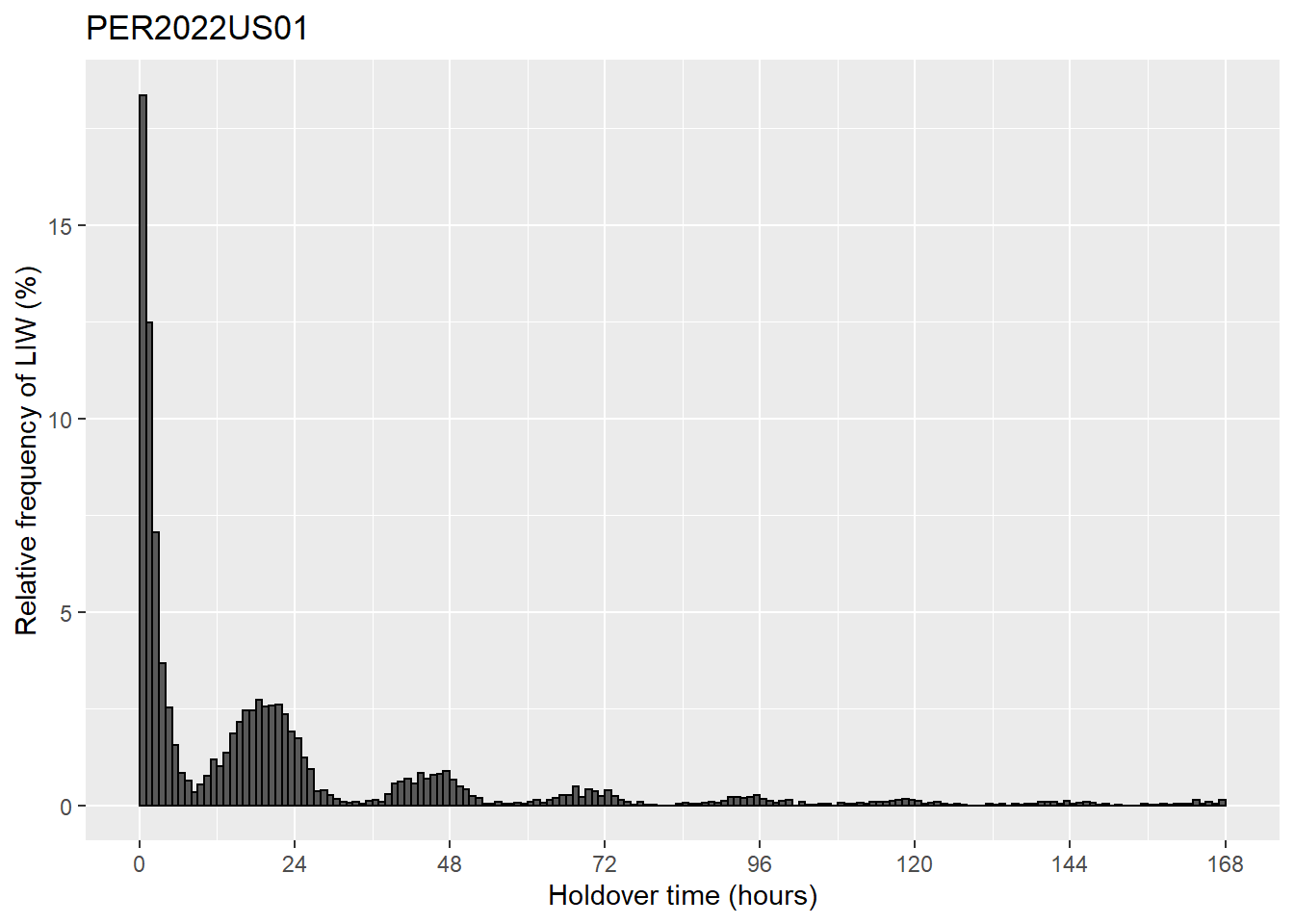ggplot(h1, aes(x=HOT, y=..count../sum(..count..)*100)) + # daily histogram geom_histogram(colour="black", boundary = 0, binwidth = 24) + scale_x_continuous(breaks=c(0, 24, 48, 72, 96, 120, 144, 168)) + labs(x = "Holdover time (hours)", y = "Relative frequency of LIW (%)") + ggtitle(h1$Study_id) +
stat_bin(binwidth = 24, geom="text", colour="black", size=3, boundary = 0,
aes(label=round(..count../sum(..count..)*100, 1)), vjust=-0.5)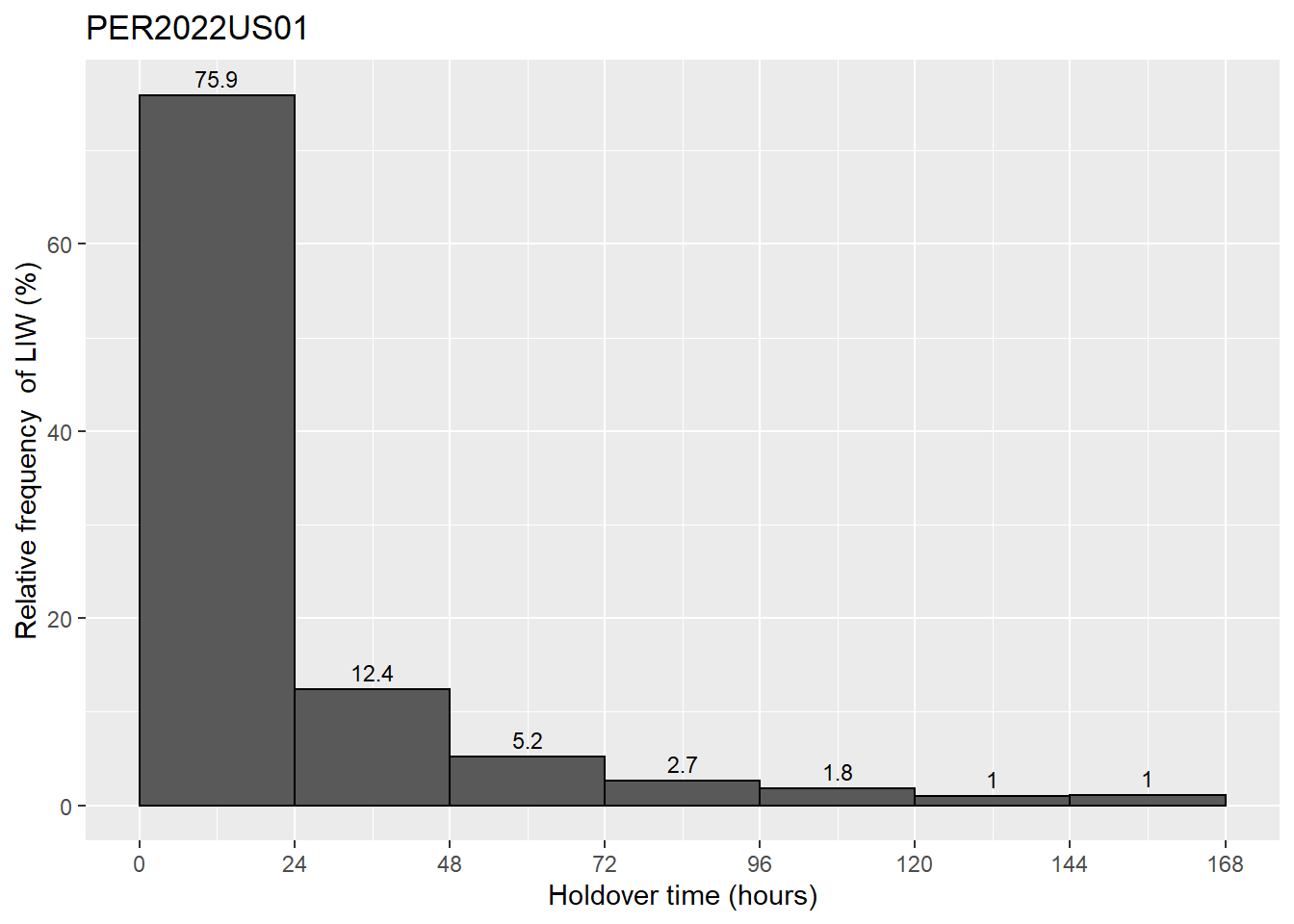## Ancillary data

### Filtering

The records of ancillary data can be filtered to select particular studies based on their characteristics. This can be done easily with the function dplyr::filter.

# check
class(AD)
##  "data.frame"
names(AD)
##   "Study_id"           "Reference"          "Publication_type"
##   "Study_area"         "Country"            "ISO_code"
##   "Spatial_scale"      "Biome"              "Ecozone"
##  "Climate_class"      "Start_year"         "End_year"
##  "Length_year"        "Min_time_h"         "Max_time_h"
##  "Number_fires"       "Number_records"     "Fire_detection"
##  "Fire_data_source"   "LLS"                "Lightning_level"
##  "DE_pct"             "LA_km"              "Method"
##  "Buffer_distance_km" "Temporal_window_d"  "Max_holdover_d"
##  "Selection_criteria" "Dataset"            "Data_collector"
##  "Date_entry"         "Data_check"         "Comments"
str(AD)
## 'data.frame':    42 obs. of  33 variables:
##  $Study_id : chr "CON2006CH01" "CON2006IT01" "KOU1967CA01" "NAS1996CA01" ... ##$ Reference         : chr  "Conedera et al 2006" "Conedera et al 2006" "Kourtz 1967" "Nash and Johnson 1996" ...
##  $Publication_type : chr "Proceeding" "Proceeding" "Report" "Paper" ... ##$ Study_area        : chr  "Ticino" "Aosta Valley" "Canada" "Alberta and Saskatchewan" ...
##  $Country : chr "Switzerland" "Italy" "Canada" "Canada" ... ##$ ISO_code          : chr  "CH-TI" "IT-23" "CA" "CA-AB; CA-SK" ...
##  $Spatial_scale : chr "Local" "Local" "Continental" "Regional" ... ##$ Biome             : chr  "Temperate coniferous forests" "Temperate coniferous forests" "Boreal forests" "Boreal forests" ...
##  $Ecozone : chr "Palearctic" "Palearctic" "Nearctic" "Nearctic" ... ##$ Climate_class     : chr  "Dfb: cold; no dry season; warm summer " "Dfb: cold; no dry season; warm summer " "Dfc: cold; no dry season; cold summer" "Dfc: cold; no dry season; cold summer" ...
##  $Start_year : int 1981 2003 1960 1988 1992 2012 2017 2000 2004 2009 ... ##$ End_year          : int  2004 2003 1963 1993 2001 2015 2017 2009 2009 2014 ...
##  $Length_year : int 24 1 4 4 10 4 1 10 6 6 ... ##$ Min_time_h        : num  24 24 4 24 24 24 24 24 0.1 0.02 ...
##  $Max_time_h : int 24 24 24 24 24 24 24 2088 24 24 ... ##$ Number_fires      : int  154 25 3615 2551 5169 797 95 1797 464 357 ...
##  $Number_records : int 7 6 16 15 28 15 11 4 24 19 ... ##$ Fire_detection    : chr  "Fire database" "Fire database" "Fire database" "Fire database" ...
##  $Fire_data_source : chr "Swissfire" "Aosta Valley wildfire database" "Survey of lightning-caused forest fire reports of Canada" "Alberta wildfire database; Saskatchewan wildfire database" ... ##$ LLS               : chr  "" "" "" "LLP" ...
##  $Lightning_level : chr "" "" "" "Flash" ... ##$ DE_pct            : chr  "" "" "" "90" ...
##  $LA_km : chr "" "" "" "5-10" ... ##$ Method            : chr  "Storm time" "Storm time" "Storm time" "Lightning match" ...
##  $Buffer_distance_km: chr "" "" "" "5" ... ##$ Temporal_window_d : chr  "" "" "" "15" ...
##  $Max_holdover_d : chr "7" "6" "> 11" "15" ... ##$ Selection_criteria: chr  "" "" "" "Minimum holdover time" ...
##  $Dataset : int 1 1 1 1 1 1 1 1 1 1 ... ##$ Data_collector    : chr  "Jose V. Moris" "Jose V. Moris" "Jose V. Moris" "Jose V. Moris" ...
##  $Date_entry : chr "3/4/2021" "3/4/2021" "3/4/2021" "10/4/2021" ... ##$ Data_check        : chr  "Yes" "Yes" "No" "No" ...
##  $Comments : chr "Data provided by G.B. Pezzatti and M. Conedera" "Data provided by G.B. Pezzatti and M. Conedera" "" "" ... dim(AD) ##  42 33 # filtering fd_it_es <- AD %>% dplyr::filter(Country %in% c("Italy", "Spain")) # studies from Italy and Spain fd_y2000 <- AD %>% dplyr::filter(Start_year >= 2000) # studies from 2000 onwards fd_200fires <- AD %>% dplyr::filter(Number_fires >= 200) # studies with at least 200 fires fd_max_prox <- AD %>% dplyr::filter(Selection_criteria == "Maximum proximity index") # studies that used the maximum proximity index fd_boreal_5y <- AD %>% dplyr::filter(Biome == "Boreal forests" & # studies from boreal regions and at least 5 years of duration Length_year >= 5) ### Data manipulation Censored, non-censored, and ancillary data can be combined to perform more analyses. We are including here a couple of examples. cd_country <- CD %>% left_join(AD %>% select(Study_id, Country), # add country information to censored data by = "Study_id") bp_ch <- cd_country %>% filter(Country == "Switzerland") %>% # filter studies from Switzerland mutate(day = cut(Upper_limit_d, # calculate daily relative frequency seq(0, max(Upper_limit_d, na.rm = T) +1))) %>% group_by(Study_id, day) %>% summarize(RF = sum(RF)) bp_ch %>% group_by(Study_id) %>% summarize(check = sum(RF)) # the values must be 1 ## # A tibble: 2 x 2 ## Study_id check ## <chr> <dbl> ## 1 CON2006CH01 1.00 ## 2 MOR2020CH01 1.00 ggplot(bp_ch, aes(x = day, y = RF*100)) + # barplot of Swiss distributions geom_bar(stat="identity") + labs(x = "Holdover time (days)", y = "Relative frequency of LIW (%)") + geom_text(aes(label = round(RF*100, 1), vjust = -0.25)) + ylim(0,80) + facet_wrap(~Study_id, ncol = 2)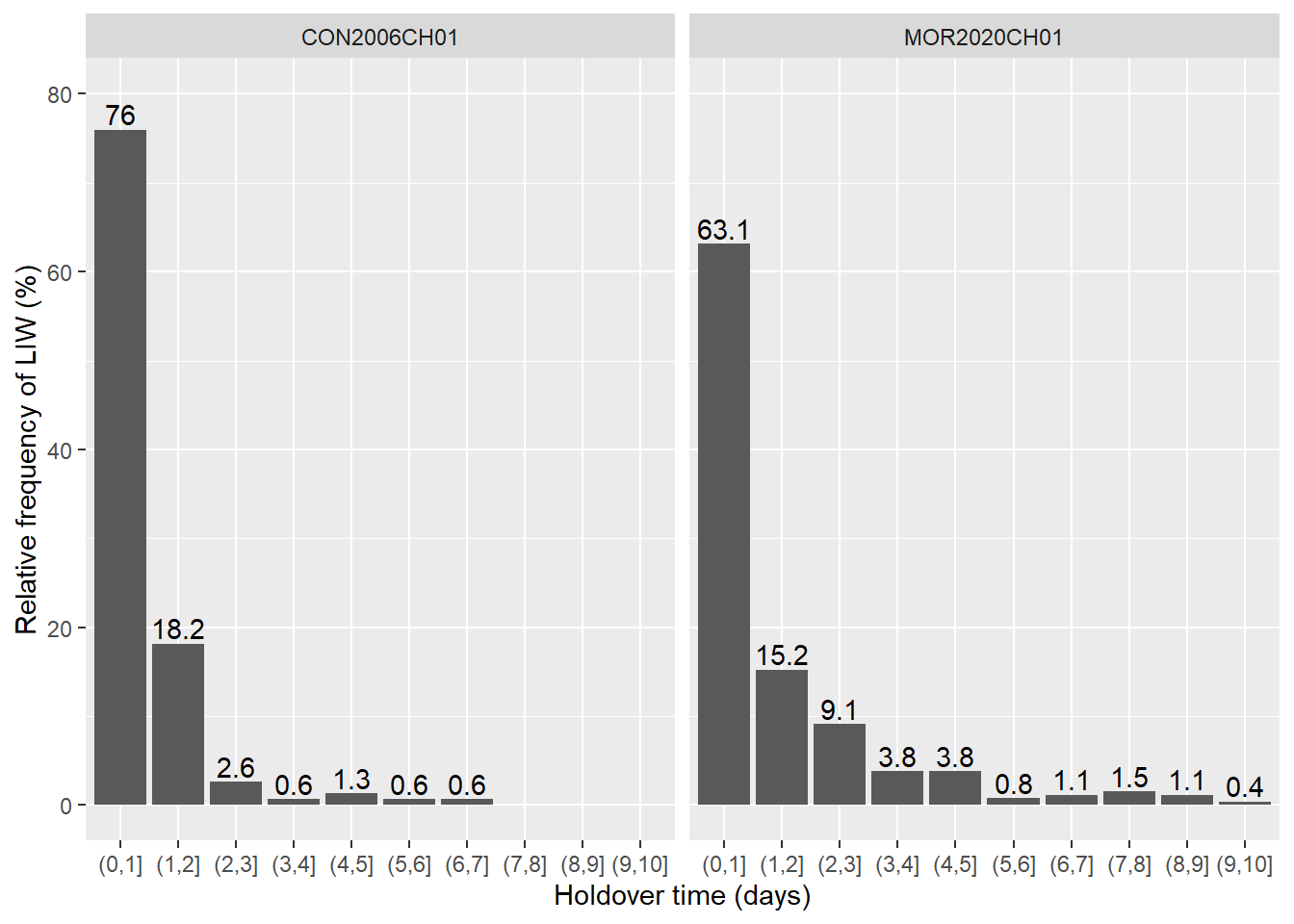ad <- AD %>% left_join(cd_sta, by = "Study_id") # add statistics calculated from censored data to ancillary data ad$Biome <- factor(ad\$Biome, # reorder biomes
levels = c("Boreal forests",
"Temperate coniferous forests",
"Mediterranean forests",
"Flooded grasslands and savannas"))

ggplot(ad, aes(x=Biome, y=median_h)) + # barplot of median HOT by biome
geom_boxplot() +
stat_boxplot(geom ="errorbar", width = 0.25) +
geom_point(color="black", shape=16) +
labs(x = "Biome",
y = "Median holdover time (hours)") +
scale_x_discrete(labels = function(x) str_wrap(x, width = 20))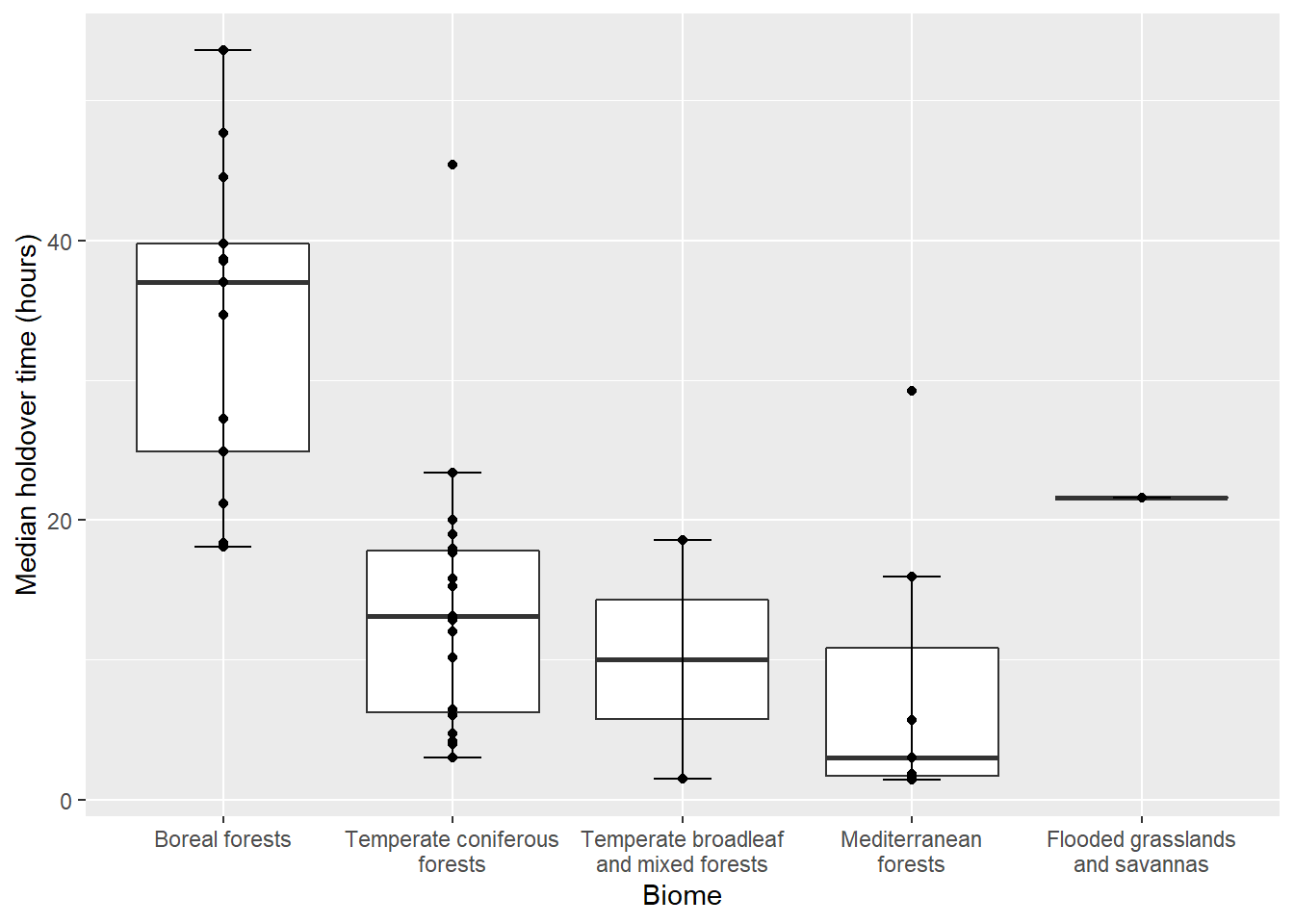# end of R script